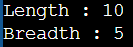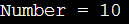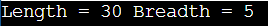# This Pointer in C++

## This Pointer in C++ with Examples:

In this article, I am going to discuss This Pointer in C++ with Examples. Please read our previous article where we discussed Inline Functions in C++ with Examples.

##### What is this Pointer in C++?

Every object in C++ has access to its own address through an important pointer called this pointer. The “this” pointer is an implicit parameter to all member functions. Therefore, inside a member function or constructor, this may be used to refer to the invoking object. So, the “this” pointer holds the address of the current object. In simple words, we can say that this pointer points to the current object of the class. We can use this pointer in the following scenarios.

1. It can be used to pass the current object as a parameter to another method.
2. It can be used to refer current class instance variable.
3. It can be used to declare indexers.

Note: Friend functions do not have this pointer, because friends are not members of a class. Only member functions including constructors have “this” pointer.

Syntax to use this pointer in C++:
this
this->member-identifier

##### Using this pointer inside Constructor in C++

Let us understand this pointer with an example. Please have a look at the below code. Here we have defined a class called Rectangle. This class is having length and breadth as private data members. Then we have also defined two constructors and two setter and getter methods. The first constructor is taking two parameters i.e. l for length and b for breadth. And the second constructor is a copy constructor.

```class Rectangle
{
private:
int length;
public:
Rectangle (int l, int b)
{
length = l;
}
Rectangle (Rectangle & rect)
{
length = rect.length;
}
void setLength (int l)
{
if (l >= 0)
length = l;
else
length = 0;
}
{
if (b >= 0)
else
}
int getLength ()
{
return length;
}
{
}
};
```

Now let us discuss ‘this’ pointer. Suppose we modify the parameters of the first constructor as l to length and b to breadth as follows.
Rectangle(int length, int breadth){ … }

Then inside this constructor we will assign length and breadth as follows:
length = length;
}
Now, we will be confused. Now, we cannot tell which length variable belongs to class and which length variable belongs to function, and also the same for the breadth variable.

##### How we will differentiate between these two length and two breadth variables?

Actually, we want to store the value of the parameter’s length and breadth value into the class’s data member length and breadth. So how to refer to data members of the same class or the same object? So, to avoid the name ambiguity and to make the statement clearer, we can use this pointer as follows,

this->length = length;
}

The ‘this’ is a pointer to the current object or the same object in C++. So ‘this->length’ is referring to the class’s length variable and ‘length’ refers to function parameter length. Same with breadth. Now, let us write the main function.

int main(){
Rectangle r(10, 5);
return 0;
}

Now inside the main function, we have created an object r of class Rectangle. Here we have passed 10 and 5 in the constructor and these values will be assigned to the object’s length and breadth data members.

##### Example to Understand This Pointer in C++ inside Constructor:
```#include <iostream>
using namespace std;
class Rectangle
{
private:
int length;
public:
{
this->length = length;
}
Rectangle (Rectangle & rect)
{
length = rect.length;
}
void setLength (int l)
{
if (l >= 0)
length = l;
else
length = 0;
}
{
if (b >= 0)
else
}
int getLength ()
{
return length;
}
{
}
};
int main(){
Rectangle r(10, 5);
cout<<"Length : "<<r.getLength()<<endl;
return 0;
}
```
###### Output:##### Example: local variable is the same as a member’s name

When the local variable’s name is the same as the class member’s name, then by default the local variable will hide the class variable. In that case, we can use the ‘this’ pointer to retrieve the object’s member which is hidden by the local variable. In the below example, we declare the private class member number, and also you notice that in the setNumber method the parameter name is also number. This parameter number will hind the class number variable. So, to refer to the class number variable, here we are using this pointer like this->number.

```#include<iostream>
using namespace std;
class Test
{
private:
int number;
public:
void setNumber(int number)
{
// The 'this' pointer is used to retrieve the object's number hidden by the local variable number
this->number = number;
}
void Display()
{
cout << "Number = " << number << endl;
}
};

int main ()
{
Test obj;
int num = 10;
obj.setNumber(num);
obj.Display();
return 0;
}
```
###### Output:##### Example: This Pointer in C++ returns a reference to the calling object

We can also return a reference to the calling object by using the “this” operator. The syntax is given below.

Test& Test::func()
{
// Some processing
return *this;
}

Another example of using this pointer is to return the reference of the current object so that you can chain function calls, this way you can call all the functions for the current object in one go. That means when a reference to a local object is returned, the returned reference can be used to chain function calls on a single object. Let us understand this with an example.

```#include<iostream>
using namespace std;

class Rectangle
{
private:
int length;
public:
Rectangle(int length = 0, int breadth = 0)
{
this->length = length;
}
Rectangle& setLength(int a)
{
length = a;
return *this;
}
{
return *this;
}
void Display()
{
cout << "Length = " << length << " Breadth = " << breadth << endl;
}
};

int main ()
{
Rectangle rect(10, 5);

// Chained function calls. All calls modify the same object as the same object is returned by reference

rect.Display();
return 0;
}
```
###### Output:Note: The “this” pointer is a pointer accessible only within the non-static member functions of a class, struct, or union type. It points to the object for which the member function is called. Static member functions don’t have a “this” pointer.

1.